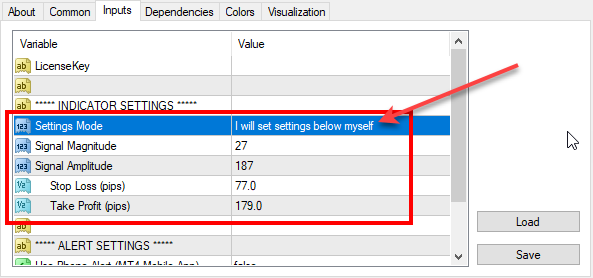# Re-optimized FxMagnetic indicator on GBPUSD (2019-10-01)

We’ve reoptimized FxMagnetic indicator settings on GBPUSD. Here are the latest parameters for all GBPUSD timeframes that you should use for best MT4 indicator performance.To set parameters manually you need to switch the Settings Mode to: I will set settings below myself

Don’t have FxMagnetic indicator yet? You can buy FxMagnetic here.

## 1-minute chart:

Signal Magnitude = 30

Signal Amplitude = 225

Stop Loss (pips) = 20

Take Profit (pips) = 59

## 5-minute chart:

Signal Magnitude = 40

Signal Amplitude = 119

Stop Loss (pips) = 84

Take Profit (pips) = 108

## 15-minute chart:

Signal Magnitude = 60;

Signal Amplitude = 251;

Stop Loss (pips) = 91;

Take Profit (pips) = 142;

## 30-minute chart:

Signal Magnitude = 30;

Signal Amplitude = 201;

Stop Loss (pips) = 112;

Take Profit (pips) = 203;

## 1-hour chart:

Signal Magnitude = 92;

Signal Amplitude = 170;

Stop Loss (pips) = 113;

Take Profit (pips) = 174;

## 4-hour chart:

Signal Magnitude = 10;

Signal Amplitude = 123;

Stop Loss (pips) = 142;

Take Profit (pips) = 213;

## Daily chart:

Signal Magnitude = 10;

Signal Amplitude = 112;

Stop Loss (pips) = 175;

Take Profit (pips) = 241;

## Weekly chart:

Signal Magnitude = 2;

Ind0Param1 = 100;

Stop Loss (pips) = 1500;

Take Profit (pips) = 500;

## Monthly chart:

Signal Magnitude = 2;

Signal Amplitude = 100;

Stop Loss (pips) = 1500;

Take Profit (pips) = 1000;

## FxMagnetic GBPUSD v1.3 stats on 1st October, 2019

Below are the screenshots of FxMagnetic GBPUSD stats from the previous 100 signals. This was taken on 2019-10-01 on FxOpen Live ECN account. We re-optimized FxMagnetic GBPUSD v1.3 on this broker so you’ll see best signals with them.

Remember, past performance does not guarantee future results.

We do not know the future and none of world’s trading algorithms know the future. All the stats above and trading signals are based on pure mathematical formulas and probability theory.